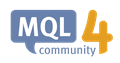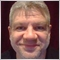# How can one create an MQL4 function that accepts a parameter that is an array whose elements' datatype is unknown?59

Hello,

How can one create an MQL4 function that accepts a parameter that is an array whose elements' datatype is unknown, such as MQL4's built-in ArraySize function?

https://docs.mql4.com/array/arraysize

int  ArraySize(
const void&  array[]    // checked array
);

The ArraySize function is really flexible because one can use it to find the total number of elements in an array of any type, such as double[], int[], string[], etc.

I am in the process of creating many array functions which call all the built-in MQL4 array functions which accept arrays of unknown datatypes, and it will be a bit of a nightmare if I have to create overloaded versions that differ only by the array datatype.

What is the MQL4 syntax for doing this?

Note that copying the syntax of ArraySize shown above doesn't work.  For example, the code below...

int MyFunction_That_Accepts_An_Array_Of_Unknown_Element_DataType(const void& array[])
{
return 1;
}

... causes a compilation error that says "'const' - illegal use of 'void'"

Thank you very much in advance,

RoboxArraySize - Array Functions - MQL4 Reference
• docs.mql4.com
ArraySize - Array Functions - MQL4 Reference42593

Robox:

Hello,

How can one create an MQL4 function that accepts a parameter that is an array whose elements' datatype is unknown, such as MQL4's built-in ArraySize function?

https://docs.mql4.com/array/arraysize

int  ArraySize(
const void&  array[]    // checked array
);

The ArraySize function is really flexible because one can use it to find the total number of elements in an array of any type, such as double[], int[], string[], etc.

I am in the process of creating many array functions which call all the built-in MQL4 array functions which accept arrays of unknown datatypes, and it will be a bit of a nightmare if I have to create overloaded versions that differ only by the array datatype.

What is the MQL4 syntax for doing this?

Note that copying the syntax of ArraySize shown above doesn't work.  For example, the code below...

int MyFunction_That_Accepts_An_Array_Of_Unknown_Element_DataType(const void& array[])
{
return 1;
}

... causes a compilation error that says "'const' - illegal use of 'void'"

Thank you very much in advance,

Robox

You can't use void.

Use a template.

template <typename T>
int MyFunction_That_Accepts_An_Array_Of_Unknown_Element_DataType(const T &array[])
{
return 1;
}59

Alain Verleyen:

You can't use void.

Use a template.

template <typename T>
int MyFunction_That_Accepts_An_Array_Of_Unknown_Element_DataType(const T &array[])
{
return 1;
}

Thanks, Alain!  It works perfectly!  This is actually so much more useful than if MQL4 supported user-programmed functions with "void& array[]" params.  I am going to use templates quite a bit going forward to write less, more maintainable code.

Thanks again!

Robox